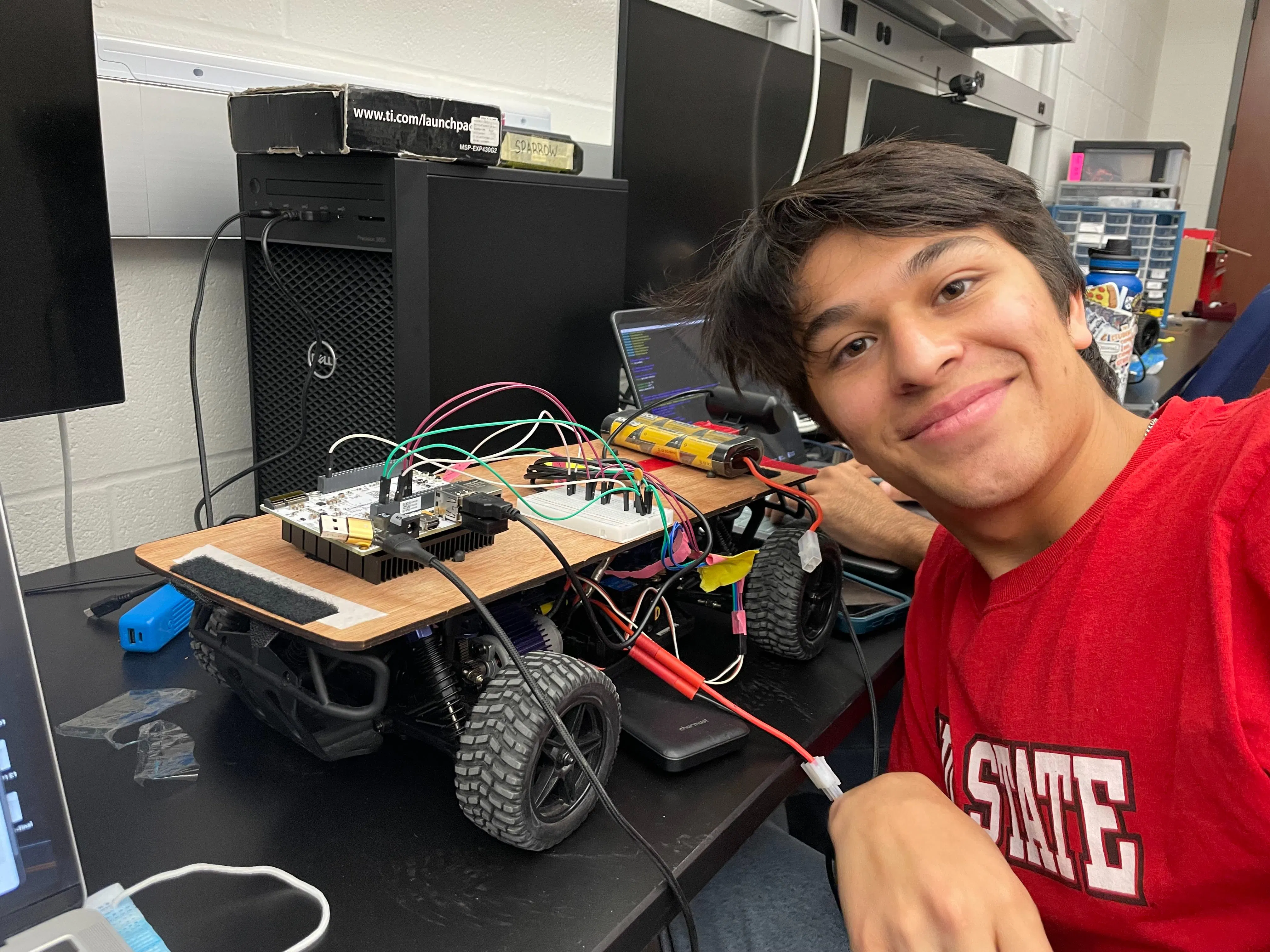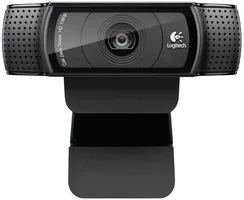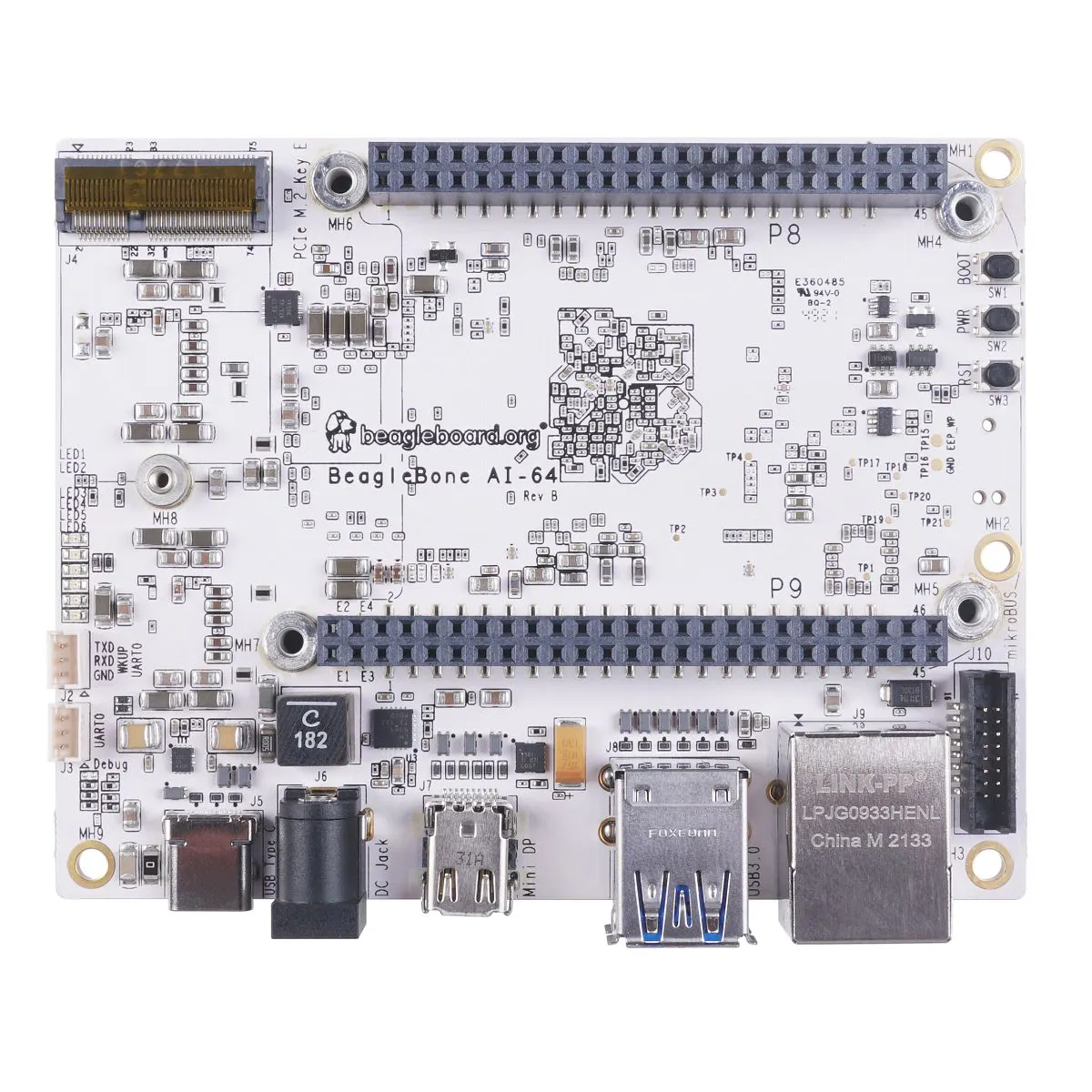Published

# Coming Soon

Our project is an autonomous car that will use lane tracking to follow a path and also stop in areas where the path is red.

BeginnerShowcase (no instructions)13## Things used in this project

### Hardware componentsWebcam, Logitech® HD Pro
×1BeagleBoard.org BeagleBone AI-64
×1
×1
 Portable Charger Power Bank
×1

### Software apps and online servicesOpenCV

## Code

### work.py

Python
Our final working algorithm to run our autonomous vehicle.
```# Uses code from https://www.instructables.com/Autonomous-Lane-Keeping-Car-Using-Raspberry-Pi-and/ and from https://www.hackster.io/really-bad-idea/autonomous-path-following-car-6c4992

import cv2
import numpy as np
import matplotlib.pyplot as plt
import math
import time

def getRedFloorBoundaries():
"""
Gets the hsv boundaries and success boundaries indicating if the floor is red
:return: [[lower color and success boundaries for red floor], [upper color and success boundaries for red floor]]
"""
return getBoundaries("redboundaries.txt")

def isRedFloorVisible(frame):
"""
Detects whether or not the floor is red
:param frame: Image
:return: [(True is the camera sees a red on the floor, false otherwise), video output]
"""
#print("Checking for floor stop")
boundaries = getRedFloorBoundaries()
return isMostlyColor(frame, boundaries)

def isMostlyColor(image, boundaries):
"""
Detects whether or not the majority of a color on the screen is a particular color
:param image:
:param boundaries: [[color boundaries], [success boundaries]]
:return: boolean if image satisfies provided boundaries, and an image used for debugging
"""
#Convert to HSV color space
hsv_img = cv2.cvtColor(image, cv2.COLOR_BGR2HSV)
#print(hsv_img[len(hsv_img) // 2, len(hsv_img) // 2])
#parse out the color boundaries and the success boundaries
color_boundaries = boundaries
percentage = boundaries

lower = np.array(color_boundaries)
upper = np.array(color_boundaries)

#Calculate what percentage of image falls between color boundaries
#print("percentage_detected " + str(percentage_detected) + " lower " + str(lower) + " upper " + str(upper))
# If the percentage percentage_detected is betweeen the success boundaries, we return true, otherwise false for result
result = percentage < percentage_detected <= percentage
#if result:
print(percentage_detected)
return result, output

def getBoundaries(filename):
"""
Reads the boundaries from the file filename
Format:
 lower: [H, S, V, lower percentage for classification of success]
 upper: [H, S, V, upper percentage for classification of success]
:param filename: file containing boundary information as above
:return: [[lower color and success boundaries], [upper color and success boundaries]]
"""
default_lower_percent = 50
default_upper_percent = 100
with open(filename, "r") as f:
lower_data = [val for val in boundaries.split(",")]
upper_data = [val for val in boundaries.split(",")]

if len(lower_data) >= 4:
lower_percent = float(lower_data)
else:
lower_percent = default_lower_percent

if len(upper_data) >= 4:
upper_percent = float(upper_data)
else:
upper_percent = default_upper_percent

lower = [int(x) for x in lower_data[:3]]
upper = [int(x) for x in upper_data[:3]]
boundaries = [lower, upper]
percentages = [lower_percent, upper_percent]
return boundaries, percentages

def stop():
"""
Stops the car
:return: none
"""
with open('/dev/bone/pwm/1/a/duty_cycle', 'w') as filetowrite:
filetowrite.write(str(int(FACTOR * dont_move)))

def go():
"""
Sends the car forward at a default PWM
:return: none
"""
with open('/dev/bone/pwm/1/a/duty_cycle', 'w') as filetowrite:
filetowrite.write(str(int(FACTOR * go_forward)))

def detect_edges(frame):
# filter for blue lane lines
hsv = cv2.cvtColor(frame, cv2.COLOR_BGR2HSV)
# cv2.imshow("HSV",hsv)
lower_blue = np.array([90, 120, 0], dtype="uint8")
upper_blue = np.array([150, 255, 255], dtype="uint8")

# detect edges
# cv2.imshow("edges",edges)

return edges

def region_of_interest(edges):
height, width = edges.shape

# only focus lower half of the screen
polygon = np.array([[
(0, height),
(0, height / 2),
(width, height / 2),
(width, height),
]], np.int32)

# cv2.imshow("roi",cropped_edges)

return cropped_edges

def detect_line_segments(cropped_edges):
rho = 1
theta = np.pi / 180
min_threshold = 10

line_segments = cv2.HoughLinesP(cropped_edges, rho, theta, min_threshold,
np.array([]), minLineLength=5, maxLineGap=150)

return line_segments

def average_slope_intercept(frame, line_segments):
lane_lines = []

if line_segments is None:
print("no line segments detected")
return lane_lines

height, width, _ = frame.shape
left_fit = []
right_fit = []

boundary = 1 / 3
left_region_boundary = width * (1 - boundary)
right_region_boundary = width * boundary

for line_segment in line_segments:
for x1, y1, x2, y2 in line_segment:
if x1 == x2:
print("skipping vertical lines (slope = infinity")
continue

fit = np.polyfit((x1, x2), (y1, y2), 1)
slope = (y2 - y1) / (x2 - x1)
intercept = y1 - (slope * x1)

if slope < 0:
if x1 < left_region_boundary and x2 < left_region_boundary:
left_fit.append((slope, intercept))
else:
if x1 > right_region_boundary and x2 > right_region_boundary:
right_fit.append((slope, intercept))

left_fit_average = np.average(left_fit, axis=0)
if len(left_fit) > 0:
lane_lines.append(make_points(frame, left_fit_average))

right_fit_average = np.average(right_fit, axis=0)
if len(right_fit) > 0:
lane_lines.append(make_points(frame, right_fit_average))

return lane_lines

def make_points(frame, line):
height, width, _ = frame.shape

slope, intercept = line

y1 = height  # bottom of the frame
y2 = int(y1 / 2)  # make points from middle of the frame down

if slope == 0:
slope = 0.1

x1 = int((y1 - intercept) / slope)
x2 = int((y2 - intercept) / slope)

return [[x1, y1, x2, y2]]

def display_lines(frame, lines, line_color=(0, 255, 0), line_width=6):
line_image = np.zeros_like(frame)

if lines is not None:
for line in lines:
for x1, y1, x2, y2 in line:
cv2.line(line_image, (x1, y1), (x2, y2), line_color, line_width)

line_image = cv2.addWeighted(frame, 0.8, line_image, 1, 1)

return line_image

def display_heading_line(frame, steering_angle, line_color=(0, 0, 255), line_width=5):
height, width, _ = frame.shape

steering_angle_radian = steering_angle / 180.0 * math.pi

x1 = int(width / 2)
y1 = height
x2 = int(x1 - height / 2 / math.tan(steering_angle_radian))
y2 = int(height / 2)

cv2.line(heading_image, (x1, y1), (x2, y2), line_color, line_width)

def get_steering_angle(frame, lane_lines):
height, width, _ = frame.shape

if len(lane_lines) == 2:
_, _, left_x2, _ = lane_lines
_, _, right_x2, _ = lane_lines
mid = int(width / 2)
x_offset = (left_x2 + right_x2) / 2 - mid
y_offset = int(height / 2)

elif len(lane_lines) == 1:
x1, _, x2, _ = lane_lines
x_offset = x2 - x1
y_offset = int(height / 2)

elif len(lane_lines) == 0:
x_offset = 0
y_offset = int(height / 2)

angle_to_mid_deg = int(angle_to_mid_radian * 180.0 / math.pi)
steering_angle = angle_to_mid_deg + 90

return steering_angle

def plot_pd(p_vals, d_vals, error, show_img=False):
fig, ax1 = plt.subplots()
t_ax = np.arange(len(p_vals))
ax1.plot(t_ax, p_vals, '-', label="P values")
ax1.plot(t_ax, d_vals, '-', label="D values")
ax2 = ax1.twinx()
ax2.plot(t_ax, error, '--r', label="Error")

ax1.set_xlabel("Frames")
ax1.set_ylabel("PD Value")
ax2.set_ylim(-90, 90)
ax2.set_ylabel("Error Value")

plt.title("PD Values over time")
fig.legend()
fig.tight_layout()
plt.savefig("pd_plot.png")

if show_img:
plt.show()
plt.clf()

def plot_pwm(speed_pwms, turn_pwms, error, show_img=False):
fig, ax1 = plt.subplots()
t_ax = np.arange(len(speed_pwms))
ax1.plot(t_ax, speed_pwms, '-', label="Speed PWM")
ax1.plot(t_ax, turn_pwms, '-', label="Steering PWM")
ax2 = ax1.twinx()
ax2.plot(t_ax, error, '--r', label="Error")

ax1.set_xlabel("Frames")
ax1.set_ylabel("PWM Values")
ax2.set_ylabel("Error Value")

plt.title("PWM Values over time")
fig.legend()
plt.savefig("pwm_plot.png")

if show_img:
plt.show()
plt.clf()

with open('/dev/bone/pwm/1/a/duty_cycle', 'w') as filetowrite:
filetowrite.write('1500000')
input()
with open('/dev/bone/pwm/1/b/duty_cycle', 'w') as filetowrite:
filetowrite.write('1500000')

FACTOR = 20000000 / 100

# Going
go_forward = 8.0
dont_move = 7.5

# Steering
left = 9
right = 6

# Max number of loops
max_ticks = 2000

# Booleans for handling stop light
passedStopLight = False
atStopLight = False
passedFirstStopSign = False

secondStopLightTick = 0

# set up video
video = cv2.VideoCapture(2)
video.set(cv2.CAP_PROP_FRAME_WIDTH, 320)
video.set(cv2.CAP_PROP_FRAME_HEIGHT, 240)

# wait for video to load
time.sleep(1)

# PD variables
kp = 0.080
kd = kp * 0.15
lastTime = 0
lastError = 0

# counter for number of ticks
counter = 0

# start the engines
go()

# arrays for making the final graphs
p_vals = []
d_vals = []
err_vals = []
speed_pwm = []
steer_pwm = []
current_speed = go_forward

sightDebug = True

stopSignCheck = 1
isStopSignBool = False
passedStopLight = False
stopSignTick = 0
stopSignCheck = 1
turnRightCount = 0
stopSignCount = 0
while counter < max_ticks:
frame = cv2.resize(original_frame, (160, 120))
if sightDebug:
cv2.imshow("Resized Frame", frame)

# check for stop sign/traffic light every couple ticks
if ((counter + 1) % stopSignCheck) == 0:
if counter > stopSignTick:
isStopSignBool, floorSight = isRedFloorVisible(frame)

if sightDebug:
cv2.imshow("floorSight", floorSight)

# If a stop sign is detected, then stop it and sleep for 2 seconds
if isStopSignBool:
stopSignCount += 1
time.sleep(0.10)
print("Detected stop sign, stopping now.")
stop()
time.sleep(2)
stopSignTick = counter + 200
passedStopLight = True
print("Stop sign finished.")
if stopSignCount == 2:
break

# If the car has just passed a stop light, then it goes again
if passedStopLight:
go()
passedStopLight = False

# process the frame to determine the desired steering angle
# cv2.imshow("original",frame)
edges = detect_edges(frame)
roi = region_of_interest(edges)
line_segments = detect_line_segments(roi)
lane_lines = average_slope_intercept(frame, line_segments)
lane_lines_image = display_lines(frame, lane_lines)
steering_angle = get_steering_angle(frame, lane_lines)

# calculate changes for PD
now = time.time()
dt = now - lastTime
if sightDebug:
cv2.imshow("Cropped sight", roi)
deviation = steering_angle - 90

# PD Code
error = -deviation
base_turn = 7.5
proportional = kp * error
derivative = kd * (error - lastError) / dt

# take values for graphs
p_vals.append(proportional)
d_vals.append(derivative)
err_vals.append(error)

# determine actual turn to do
turn_amt = base_turn + proportional + derivative
# caps turns to make PWM values
if 7.2 < turn_amt < 7.8:
print("Keep STRAIGHT")
turn_amt = 7.5
turnRightCount = 0
elif turn_amt > left:
print("Turn LEFT")
turn_amt = left
turnRightCount = 0
elif turn_amt < right:
print("Turn RIGHT")
turnRightCount += 1
turn_amt = right

# turn!
with open('/dev/bone/pwm/1/b/duty_cycle', 'w') as filetowrite:
filetowrite.write(str(int(FACTOR * turn_amt)))
if turnRightCount == 5:
time.sleep(0.25)
# take values for graphs
steer_pwm.append(turn_amt)
speed_pwm.append(current_speed)

# update PD values for next loop
lastError = error
lastTime = time.time()

key = cv2.waitKey(1)
if key == 27:
break

counter += 1

# clean up resources
video.release()
cv2.destroyAllWindows()
with open('/dev/bone/pwm/1/b/duty_cycle', 'w') as filetowrite:
filetowrite.write(str(int(FACTOR * dont_move)))
with open('/dev/bone/pwm/1/a/duty_cycle', 'w') as filetowrite:
filetowrite.write(str(int(FACTOR * dont_move)))

plot_pd(p_vals, d_vals, err_vals, True)
plot_pwm(speed_pwm, steer_pwm, err_vals, True)
```

### gpiod_driver.c

C/C++
This is our driver code to help keep a constant speed.
```//Uses code from https://www.instructables.com/Autonomous-Lane-Keeping-Car-Using-Raspberry-Pi-and/ and from https://www.hackster.io/really-bad-idea/autonomous-path-following-car-6c4992

#include <linux/module.h>
#include <linux/of_device.h>
#include <linux/kernel.h>
#include <linux/gpio/consumer.h>
#include <linux/platform_device.h>
#include <linux/init.h>
#include <linux/gpio.h>
#include <linux/interrupt.h>
#include <linux/ktime.h>

//Inturupt handler number
int irq_number;

// //Refers to LED pin P9-27
// struct gpio_desc *led;

//refers to button pin P8_14
struct gpio_desc *button;

ktime_t curr_time, previous_time;
int diff;
module_param(diff, int, S_IRUGO);

/**
* Interrupt service routine is called, when interrupt is triggered
* Got this code from https://github.com/Johannes4Linux/Linux_Driver_Tutorial/blob/main/11_gpio_irq/gpio_irq.c
*/
static irq_handler_t gpio_irq_handler(unsigned int irq, void *dev_id, struct pt_regs *regs) {
printk("Speed encoder triggered.\n");
//gpiod_set_value(led, (gpiod_get_value(led) + 1) %2);
//clock_t new_time = clock();
// if last_time == NULL {
// 	last_time = new_time;
// } else {
curr_time = ktime_get();
int temp = ktime_to_ns(curr_time - previous_time) / 1000000;
if(temp > 1) { diff = temp; }
printk("Difference between triggers: %d\n", diff);
previous_time = curr_time;
// 	last_time = current;
//     int millseconds = diff * 1000/CLOCKS_PER_SEC;
// 	printk("Milliseconds: %d", millseconds);
// 	if (millseconds > 1){
// 		FILE *fptr;
// 		fptr = fopen("speed.txt", "w");
// 		fprintf(fptr, "%d", millseconds);
// 		fclose(fptr);
// 	}
//	je = jiffies;
//      printk("This is the previous time (js): %ld\n", js);
//    printk("This is the current time (je): %ld\n", je);
//	diffj = js - je;
//	unsigned int check;
//	check  = jiffies_to_usecs(diffj);
//	if (check > 1000){
//		diff = check;
//	}
//	js = je;
//	printk("Difference in rotations is now: %d\n", diff);
return (irq_handler_t) IRQ_HANDLED;
}

// probe function, takes in the platform device that allows us to get the references to the pins
static int led_probe(struct platform_device *pdev)
{
printk("gpiod_driver has been inserted.\n");
//Get the pins based on what we called them in the device tree file:  "name"-gpios
//led = devm_gpiod_get(&pdev->dev, "led", GPIOD_OUT_LOW);
button = devm_gpiod_get(&pdev->dev, "userbutton", GPIOD_IN);

//Set waiting period before next input
gpiod_set_debounce(button, 1000000);

//Pair the button pin with an irq number
irq_number = gpiod_to_irq(button);

//Pair the number with the function that handles it
if(request_irq(irq_number, (irq_handler_t) gpio_irq_handler, IRQF_TRIGGER_RISING, "my_gpio_irq", NULL) != 0){
printk("Error!\nCan not request interrupt nr.: %d\n", irq_number);
free_irq(irq_number, NULL);
return -1;
}
return 0;
}

// remove function ,takes in the platform device that allows us to get the references to the pins
static int led_remove(struct platform_device *pdev)
{
printk("Custom gpiod_driver module has been removed and irq has been freed\n");
irq_number = gpiod_to_irq(button);
//TBH not sure what goes in the second argument
free_irq(irq_number, NULL);
return 0;
}

static struct of_device_id matchy_match[] = {
{.compatible = "gpiod_driver"},
{/* leave alone - keep this here (end node) */},
};

// platform driver object
static struct platform_driver adam_driver = {
.probe  = led_probe,
.remove  = led_remove,
.driver  = {
.name  = "The Rock: this name doesn't even matter",
.owner = THIS_MODULE,
.of_match_table = matchy_match,
},
};

MODULE_DESCRIPTION("424\'s finest");
MODULE_AUTHOR("GOAT");
```

### init_pwm.py

Python
The file allowed us to reset the PWM values between runs. Basically straightened the wheels and made the car stop
```# P9_14 - Speed/ESC
with open('/dev/bone/pwm/1/a/period', 'w') as filetowrite:
filetowrite.write('20000000')
with open('/dev/bone/pwm/1/a/duty_cycle', 'w') as filetowrite:
filetowrite.write('1550000')
with open('/dev/bone/pwm/1/a/enable', 'w') as filetowrite:
filetowrite.write('1')
# P9_16 - Steering
with open('/dev/bone/pwm/1/b/period', 'w') as filetowrite:
filetowrite.write('20000000')
with open('/dev/bone/pwm/1/b/duty_cycle', 'w') as filetowrite:
filetowrite.write('1500000')
with open('/dev/bone/pwm/1/b/enable', 'w') as filetowrite:
filetowrite.write('1')
print("init_pwn has been executed")
```

### BONE-PWM0.dts

Allowed us to use the encoder with Pin P8_03
```// SPDX-License-Identifier: GPL-2.0
/*
* Copyright (C) 2022 BeagleBoard.org - https://beagleboard.org/
*
* https://elinux.org/Beagleboard:BeagleBone_cape_interface_spec#PWM
*/

/dts-v1/;
/plugin/;

#include <dt-bindings/gpio/gpio.h>
#include <dt-bindings/board/k3-j721e-bone-pins.h>

/*
* Helper to show loaded overlays under: /proc/device-tree/chosen/overlays/
*/
&{/chosen} {
overlays {
BONE-PWM0.kernel = __TIMESTAMP__;
};
};

&bone_pwm_0 {
status = "okay";
};
&{/} {
gpio-leds {
compatible = "gpiod_driver";
userbutton-gpios = <gpio_P8_03 GPIO_ACTIVE_HIGH>;
};
};
```

### extlinux.conf

Allowed us to acess PWM
```label Linux eMMC
kernel /Image
append console=ttyS2,115200n8 earlycon=ns16550a,mmio32,0x02800000 root=/dev/mmcblk0p2 ro rootfstype=ext4 rootwait net.ifnames=0 quiet
fdtdir /
fdtoverlays /overlays/BONE-PWM0.dtbo /overlays/BONE-PWM1.dtbo /overlays/BONE-PWM2.dtbo
initrd /initrd.img
```

### Coming Soon Autonomous Vehicle Github

This is the link to our repository with all our code for the project.

## Credits

### Heather Szczesniak

1 project • 0 followers

### Marco Lagos

0 projects • 0 followers

### Bikrant Das Sharma

0 projects • 0 followers

### Shourya Munjal

0 projects • 1 follower